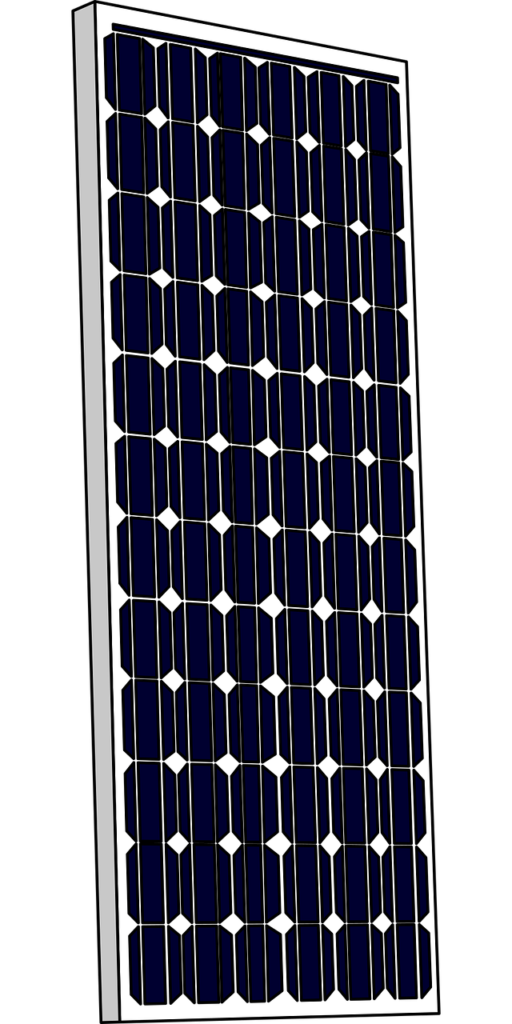# Solar PV Panel

In our previous posts we discussed about the working of a Solar PV cell and the different types of Solar PV cells and the technologies commonly available. We also saw that these cells when electrically connected in a particular arrangement result in the Solar PV Panel, commonly known as Solar PV Module. In this post we’ll now see in detail about the Solar PV Panel and its requirement.

The power of a solar cell is given by the formula, P=V x I, where P is electrical power measured in watts, V is the Voltage produced by the individual cell measured in volts and I is the output current measured in ampere. At STC, the average operating voltage of a Solar PV cell is around .5V and average output current is around 3A. Thus, the output power P is given by P=V x I which is 0.5V x 3A= 1.5 watts. So roughly the power output of a single solar cell is around 1-1.5watts at STC. This output power is not sufficient for practical uses.

So, to get solar power output for practical purposes, these individual solar cells are connected in series or parallel. When connected in series, the voltage of individual solar cells gets added up and can give us the desired voltage output and when connected in parallel, the current of individual solar cell gets added up and can give us desired current output. Hence combination of two or more solar cells are connected in series and/or in parallel to get the desired voltage, current and power rating and thus resulting into a Solar PV Panel.

Example: Let’s assume we have a requirement for a Solar PV Panel of capacity of 15 watts peak power. We have seen above that the voltage of an individual solar cell is around .5 volts and voltage get added up when solar cells are connected in series. So, we’ll connect 10 solar cells in series resulting in net voltage of .5 x 10 = 5 volts. Also, since they are connected in series their current output will be 3 amps only as the current remain constant when the solar cells are connected in series. So, the final power output in our case will be P= V x I = 5 x 3 = 15 watts peak power(Wp). Thus, we can meet our requirement of a Solar PV Panel of capacity 15 Wp.

Based on our understanding so far and the example we have seen above, the number of individual solar cell required to make a single Solar PV Panel depends on the power requirement we have, and the type of solar cells used. Solar PV Panels come in all types of capacity and size depending on our energy requirements. Commonly available Solar PV Panels come with output voltage of 12 volts and 24 volts. For a 12V output Solar PV Panel, generally there will be 36 individual solar cells connected in series and similarly for a 24V output Solar PV Panel, there will be 72 individual solar cells connected in series.We’ll see in detail about the different types of connections of solar cells to make a Solar PV Panel and their benefits and limitations in our next post.